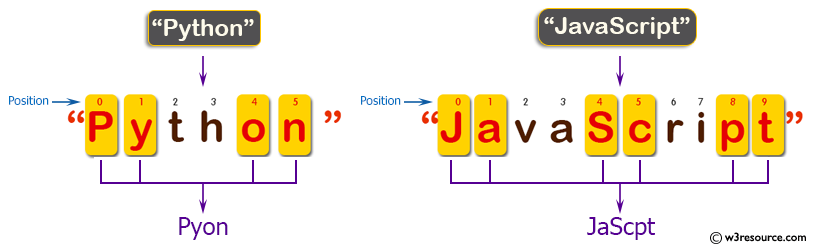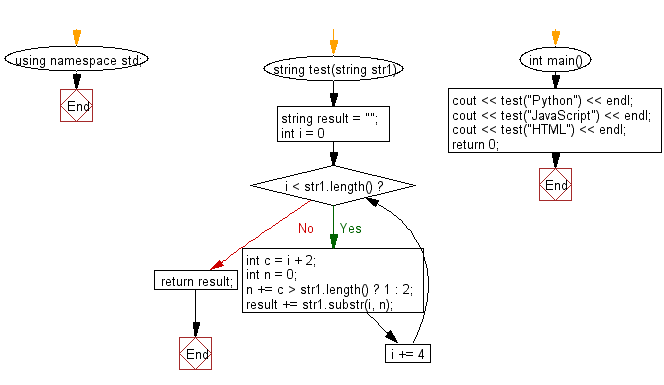﻿ C++ Exercises: Create a new string of the characters at indexes 0,1, 4,5, 8,9 ... from a given string - w3resource# C++ Exercises: Create a new string of the characters at indexes 0,1, 4,5, 8,9 ... from a given string

## C++ Basic Algorithm: Exercise-34 with Solution

Write a C++ program to create a new string of the characters at indexes 0,1, 4,5, 8,9 ... from a given string.

Sample Solution:

C++ Code :

``````#include <iostream>

using namespace std;

string test(string str1)
{
string result = "";
for (int i = 0; i < str1.length(); i += 4)
{
int c = i + 2;
int n = 0;
n += c > str1.length() ? 1 : 2;
result += str1.substr(i, n);
}
return result;
}

int main()
{
cout << test("Python") << endl;
cout << test("JavaScript") << endl;
cout << test("HTML") << endl;
return 0;
}
``````

Sample Output:

```Pyon
JaScpt
HT
```

Pictorial Presentation:Flowchart:C++ Code Editor:

Contribute your code and comments through Disqus.

What is the difficulty level of this exercise?

﻿